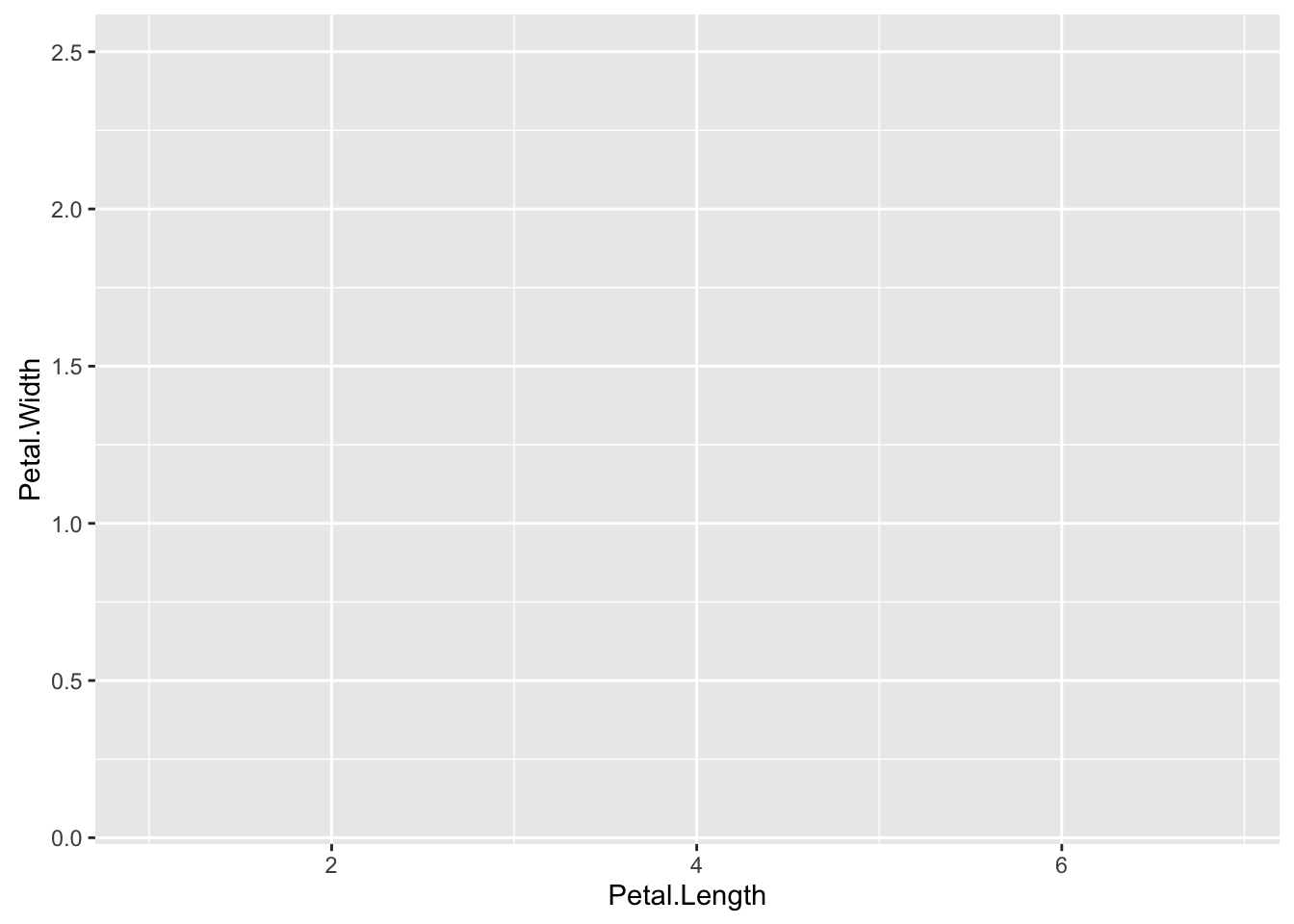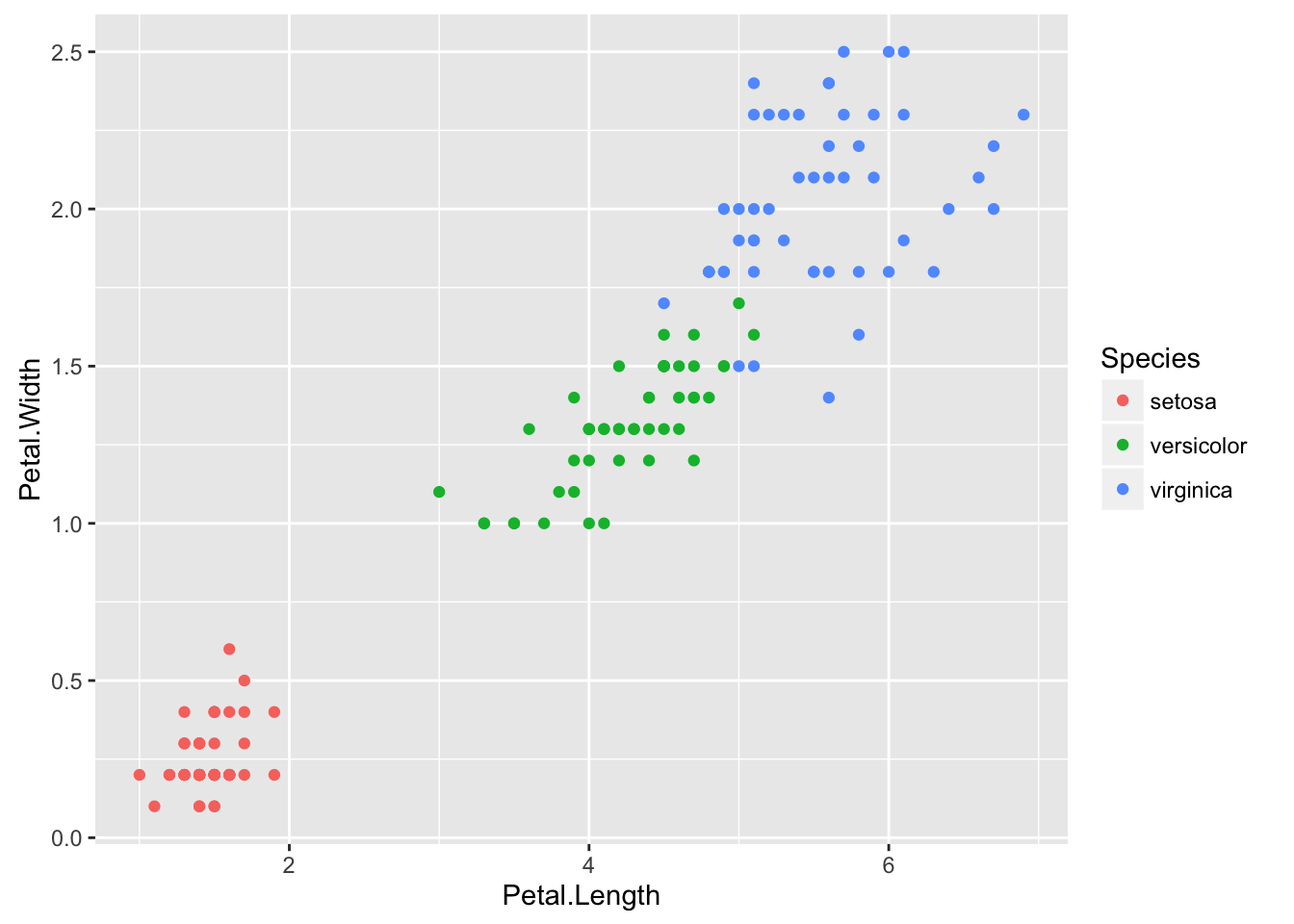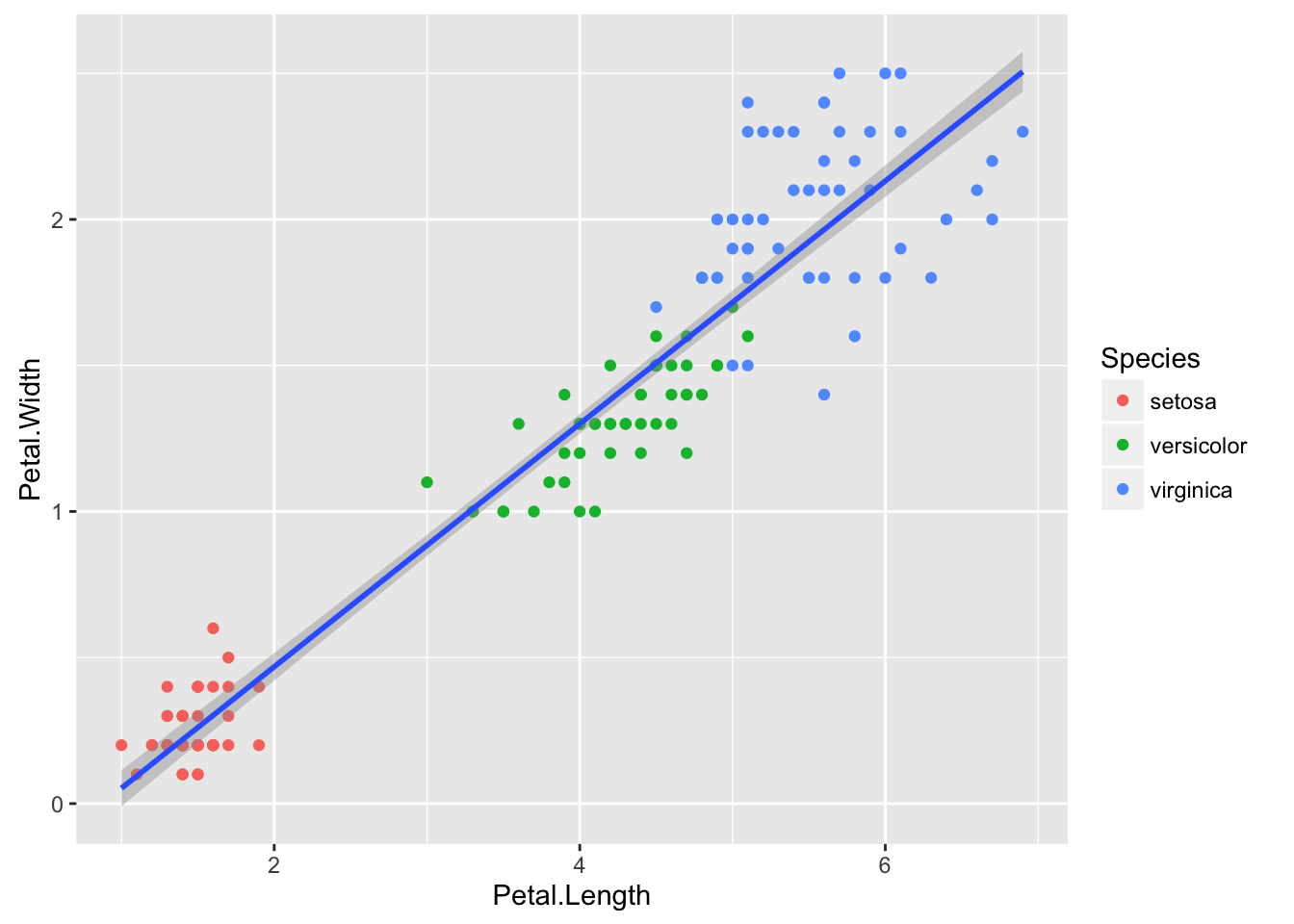This intro assumes that the readers know the basics of R. To keep everything concise, the descriptions have a tendency to be extremely short, so pointers to other references are scattered throughout this intro. To focus on presenting certain features of ggplot2, some of the graphics in this intro are not-so-ideal in the sense that better visualization can be made.

After working through this intro, you should be able to …

1. understand the key components of a ggplot plot
2. explore many other features of ggplot2 on your own.

# Motivation

## What is ggplot2?

-a data visualization package in R created by Hadley Wickham. See wikipedia.

## Why ggplot2?

1. Ability to construct plots in layers.
2. Nice looking graphics (relative to base graphics, in general).
3. Follows grammar of graphics; more intuitive.
• See the article by Hadley Wickham for more information about grammar of graphics.
1. etc.

See this github page for more reasons to use ggplot2.

# Layering

Five components of a layer:

1. Data
• a data frame containing the dataset of interest
1. Aesthetic mappings
• descriptions of how the variables in the dataset are mappeed to visual objects
• specified by the `aes()` function
1. Geom
• geometric objects
• control the type of plots
• key ones: line (lineplot), point (scatterplot), bar (barplot), histogram, density
1. Stat
• statistical transformation of the data
• minor adjustments of the position of layer elements
• mainly used for discrete data
• dodge, fill, identity, jitter, stack

We will focus on the first three in this tutorial.

## Example 1:

Using the iris dataset, create a scatterplot of petal lengths (y-axis) versus petal widths (x-axis), color coded by species. In addition, plot the regression line (petal lengths vs petal widths) with a 95% confidence band.

Let’s construct the plot step-by-step.

First, we would like to initialize our plot using `ggplot()`.

``library(ggplot2)``
``## Warning: package 'ggplot2' was built under R version 3.2.3``
``````p1 <- ggplot(data = iris, aes(x = Petal.Length, y = Petal.Width))
p1``````What does the code do? `data = iris` tells ggplot() to look at the dataset `iris`, and `aes(x = Petal.Length, y = Petal.Width))` maps `x` to the variable `Petal.Length` in `iris` and `y` to the variable `Petal.Width` in `iris` (this is evident from the x-axis and the y-axis of the above plot).

You may wonder why there is nothing shown on the plot. The reason is that we haven’t specified what we want to see on the plot! This is where `geom` comes into play.

``````p2 <- p1 + geom_point(aes(color = Species))
p2``````Three things are added to the plot:

1. Points representing the observations
2. Colors corresponding to the species
3. A legend that describes the color coding

What happened? geom_point() generates a scatterplot via a layer of points based on `x` and `y`, and `aes(color = Species)` maps `color` to the variable `Species`. One nice feature of `ggplot()` is that the legend is created automatically when color-coding/shape-coding via aesthetic mappings.

Finally, we use `geom_smooth()` with the argument `method='lm'` to plot the regression line with a confidence band.

``````p3 <- p2 + geom_smooth(method='lm')
p3``````Creating/modifying the title and the axis labels is straightforward.

``````p4 <- p3 + xlab("Petal Length (cm)") + ylab("Petal Width (cm)") + ggtitle("Petal Length versus Petal Width")
p4``````